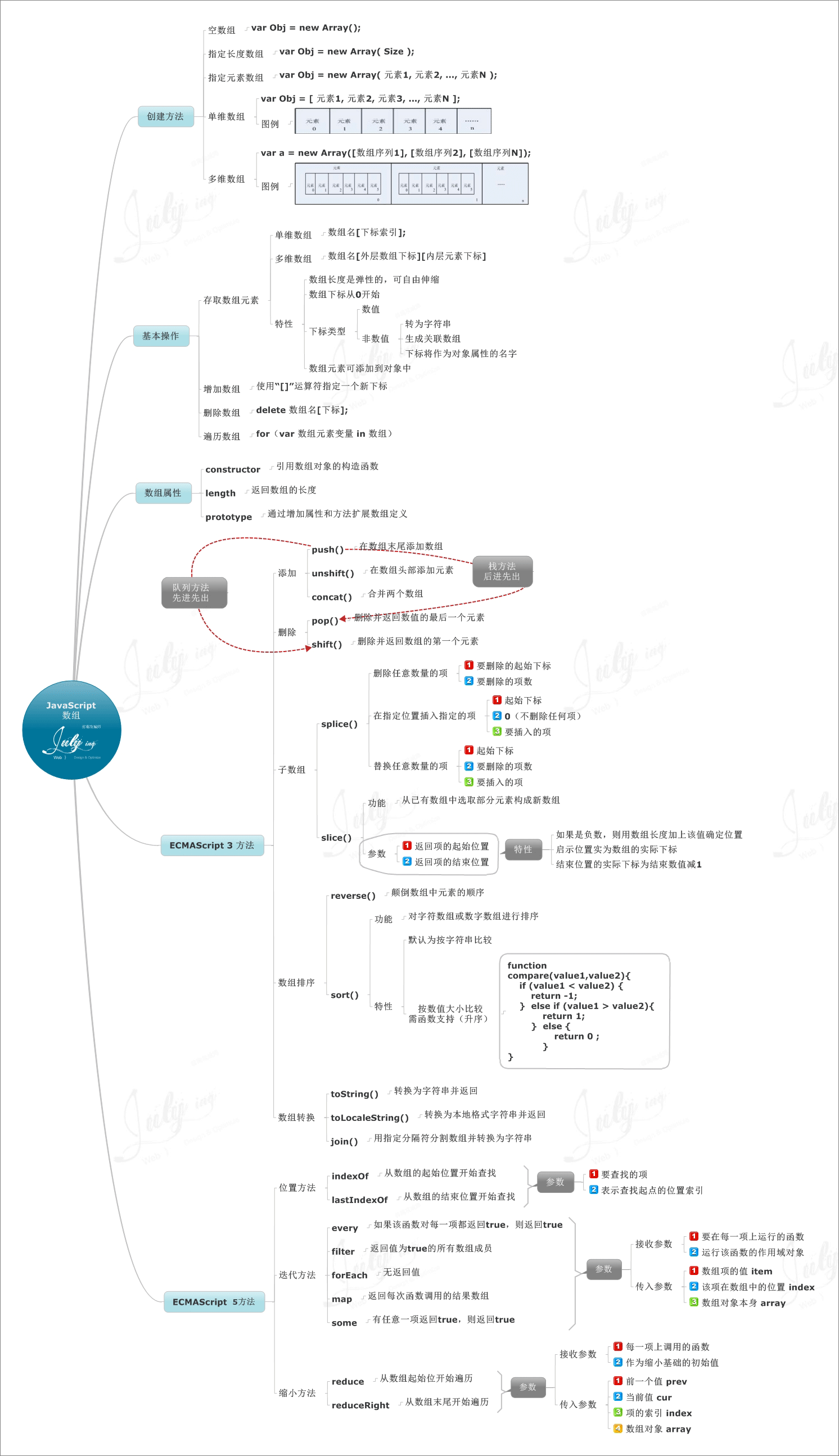# JavaScript中数组常见操作技巧

更新时间：2017年09月01日 09:21:12   作者：鲫de鱼我要评论Tip: 右键在新标签中打开查看清晰大图

```// good
var arr = [];
var arr = ['red', 'green', 'blue'];
var arr = [
['北京', 90],
['上海', 50],
['广州', 50]
];
var arr = new Object();```

```var sourceList = document.querySelector('#source');
// 取得<ul>标签下所有<li>元素
var lis = sourceList.querySelectorAll('li');
// 取得<ul>标签下所有<b>元素
var bs = sourceList.querySelectorAll('li b');
var data = [];
for (var i = 0, len = lis.length; i < len; i++) {
// 法一：先对data添加一维空数组，使其成为二维数组后继续赋值
data.push([]);
// 分割文本节点，提取城市名字
var newNod = lis[i].firstChild.splitText(2);
data[i] = lis[i].firstChild.nodeValue;
// 使用+转换数字
data[i] = +bs[i].innerHTML;
// 法二：先对一维数组赋值，再添加到data数组中，使其成为二维数组
var li = lis[i];
var city = li.innerHTML.split("空气质量：");
var num = +li.innerText.split("空气质量：");
data.push([city,num]);
}```

`String.prototype.split() `方法用于把一个字符串分割成字符串数组。 split() 方法不改变原始字符串。

`li.innerHTML.split`("空气质量：")-----这个拆成的数组为["北京"，"90"]的数组，再取数组

`Text.splitText()`方法会将一个文本节点分成两个文本节点，原来的文本节点将包含从开始到指定位置之前的内容，新文本节点将包含剩下的文本。这个方法会返回一个新文本节点

`querySelector()`方法接收一个CSS选择符，返回与改模式匹配的第一个元素，如果没有找到，则返回null

`querySelectorAll()`方法接受一个CSS选择符，返回一个NodeList对象，如果没有找到，则为空

arr[下标索引]

arr[外层数组下标][内层元素下标]

length属性

`arr[array.length] = []`

`arr.length = 0 || (少于项数的数值)`

`if(arr.length) {}`

```for(var i = 0, len = arr.length; i < len; i++){}
for...in
for (var index in arrayObj){
var obj = arrayObj[index];
}
forEach()
arr.forEach(function callback(currentValue, index, array) {
}[, thisArg]);```

```data.forEach(function (item, index) {
li = document.createElement('li');
fragment.appendChild(li);
li.innerHTML = '第' + digitToZhdigit(index + 1) + '名：' + item + '空气质量：' + '<b>' + item + '</b>';
});
const numbers = [1, 2, 3, 4];
let sum = 0;
numbers.forEach(function(numer) {
sum += number;
});
console.log(sum); ```

```var foo = true;
if(foo) {
let bar = foo*2；
bar =something(bar);
console.log(bar);
}
console.log(bar); // RefenceError```

```numbers.forEach(numer => {
});```

sort()方法

```function compare(a, b) {
if (a < b) {
return -1;
} else if (a > b) {
return 1;
} else {
return 0;
}
}```

```function compare(a, b) {
return a - b;
}```

```var objectList = [];
function Persion(name,age){
this.name=name;
this.age=age;
}
objectList.push(new Persion('jack',20));
objectList.push(new Persion('tony',25));
objectList.push(new Persion('stone',26));
objectList.push(new Persion('mandy',23));
//按年龄从小到大排序
objectList.sort(function(a,b){
return a.age-b.age
});```

```var aqiData = [
["北京", 90],
["上海", 50],
["福州", 10],
["广州", 50],
["成都", 90],
["西安", 100]
];
aqiData.sort(function (a, b) {
return a - b;
});
console.table(aqiData); // 以表格输出到控制台，用于调试直观了然```

reverse()方法

```var values = [1, 2, 3, 4, 5];
values.reverse();

```arrayObj. push([item1 [item2 [. . . [itemN ]]]]); // 将一个或多个新元素添加到数组结尾，并返回数组新长度
arrayObj.unshift([item1 [item2 [. . . [itemN ]]]]); // 将一个或多个新元素添加到数组开始，数组中的元素自动后移，返回数组新长度
arrayObj.splice(insertPos,0,[item1[, item2[, . . . [,itemN]]]]); // 将一个或多个新元素插入到数组的指定位置，插入位置的元素自动后移，返回""。第二个参数不为0（要删除的项数）时则可以实现替换的效果。
arr[array.length] = [] // 使用length属性在数组末尾添加新项```

```arrayObj.pop(); // 移除末端一个元素并返回该元素值
arrayObj.shift(); // 移除前端一个元素并返回该元素值，数组中元素自动前移
arrayObj.splice(deletePos,deleteCount); // 删除从指定位置deletePos开始的指定数量deleteCount的元素，返回所移除的元素组成的新数组```

```arrayObj.slice(startPos, [endPos]); // 以数组的形式返回数组的一部分，注意不包括 endPos 对应的元素，如果省略 endPos 将复制 startPos 之后的所有元素
arrayObj.concat([item1[, item2[, . . . [,itemN]]]]); // 将多个数组（也可以是字符串，或者是数组和字符串的混合）连接为一个数组，返回连接好的新的数组```

```arrayObj.slice(0); // 返回数组的拷贝数组，注意是一个新的数组，不是指向
arrayObj.concat(); // 返回数组的拷贝数组，注意是一个新的数组，不是指向```

```arr.indexOf(searchElement[, fromIndex = 0]) // 返回首个被找到的元素（使用全等比较符===），在数组中的索引位置; 若没有找到则返回 -1。fromIndex决定开始查找的位置，可以省略。
lastIndexOf() // 与indexOf()一样，只不过是从末端开始寻找```

`arrayObj.join(separator); //返回字符串，这个字符串将数组的每一个元素值连接在一起，中间用 separator 隔开。`

```array.reduce(function(accumulator, currentValue, currentIndex, array), initialValue）// 累加器和数组中的每个元素 (从左到右)应用一个函数，将其减少为单个值，返回函数累计处理的结果
var total = [0, 1, 2, 3].reduce(function(sum, value) {
return sum + value;
}, 0);
// total is 6```